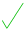From: Sam, a secondary school student If g(x) = 2x+3/5 then how do I find the inverse? Hi Sam. One way to think about finding the inverse is to think of the function as a machine, into which you put your input number, x, and out of which comes 2x + 3/5. You are asking for the reverse process. Going forward, you: (a) multiplied the input by 2 (b) then added 3/5. To reverse this (and recover the original number) you would: (c) subtract 3/5 (d) then divide by 2. As a formula, the inverse is then (y - 3/5)(1/2) You should always check your proposed answer (and there are two orders to do this in): Forwards: 2[ (y - 3/5)(1/2) ] + 3/5 = y - 3/5 + 3/5 = yand reverse: [ (2x + 3/5) - 3/5 ](1/2) = (2x + 3/5 - 3/5)(1/2) = (2x)(1/2) = xIt is good to 'make sense' out of the process, and to be able to check your conclusions. Function machines, as one of the standard 'representations' can help you do that. Walter Whiteley